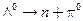Chapter 33, Problem 30PE

Chapter
Section
Textbook Problem

(a) Is the decaypossible considering the appropriate conservation laws? State why or why not.(b) Write the decay in terms of the quark constituents of the particles.

(a)

To determine

Whether the decay Λ0n+π0 is possible as per the conservation laws.

Explanation

Given info:

Given reaction is Λ0n+π0

Calculation:

We can verify whether the decay Λ0n+π0 is possible by checking the following conservation laws.

Decay:

Λ0n+π0

Charge:

0  0  0conserved

B:  +1  +1  0  conserved

Le/

To determine

(b)

To write the equation Λ0n+π0 in terms of quark structure.

Still sussing out bartleby?

Check out a sample textbook solution.

See a sample solution

The Solution to Your Study Problems

Bartleby provides explanations to thousands of textbook problems written by our experts, many with advanced degrees!

Get Started